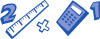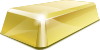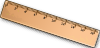* indicates a required field• phone number format invalid• email format invalidProblems detected, please review the form.
Privacy - Terms

# News from Mathnasium of Leaside

### Problem of the Week

Mar 24, 2020Lower Elementary:

Question: Start with 1. Add 15. Subtract 11. Double it. Add 1. Multiply by 5. Subtract 30. Take away half. What’s the new number?Upper Elementary:
Question: It takes Evelyn 8 minutes to jog all the way around a circular path. If Evelyn starts at 6:35 AM and jogs around the path exactly 8 times, what time will it be when she’s done?Middle School:
Question: 24–karat gold is 100% pure gold. How many grams of pure gold are there in a 6 kilogram, 16–karat gold bar?Algebra and Up:
Question: There are two perpendicular lines graphed on a plane. Line A goes through the points (2, 1) and (6, 3). Line B goes through (2, 1) and (x, 5). Solve for x.

Lower Elementary:

Solution: After we add 15 to 1, we have 16. Then we subtract 11, so we have 16 – 11 = 5. After we double it, we have 5 × 2 = 10. One more makes 11. Next, we have 11 × 5, which makes 55. Thirty less than 55 is 25. Since 25 is odd, we can find half of it by finding half of 24 and half of 1. Half of 24 is 12, and half of 1 is ½, so that gives us a final answer of 12½.

Upper Elementary:

Solution: Eight minutes, 8 times is 8 × 8 = 64 minutes. There are 60 minutes in an hour, so Evelyn jogs for 1 hour and 4 minutes because 64 minutes – 60 minutes (1 hour) = 4 minutes. One hour after 6:35 AM is 7:35 AM, and 4 minutes after that is 7:39 AM.

Middle School:

Solution:  16–karat gold is 16/24 pure gold, or 2/3. Since two thirds of the gold bar is pure gold, that means that there are 6 × 2/3 = 4 kilograms of pure gold. There are 1000 grams in a kilogram, so 4 kilograms of pure gold is the same as 4000 grams of pure gold.

Algebra and Up: